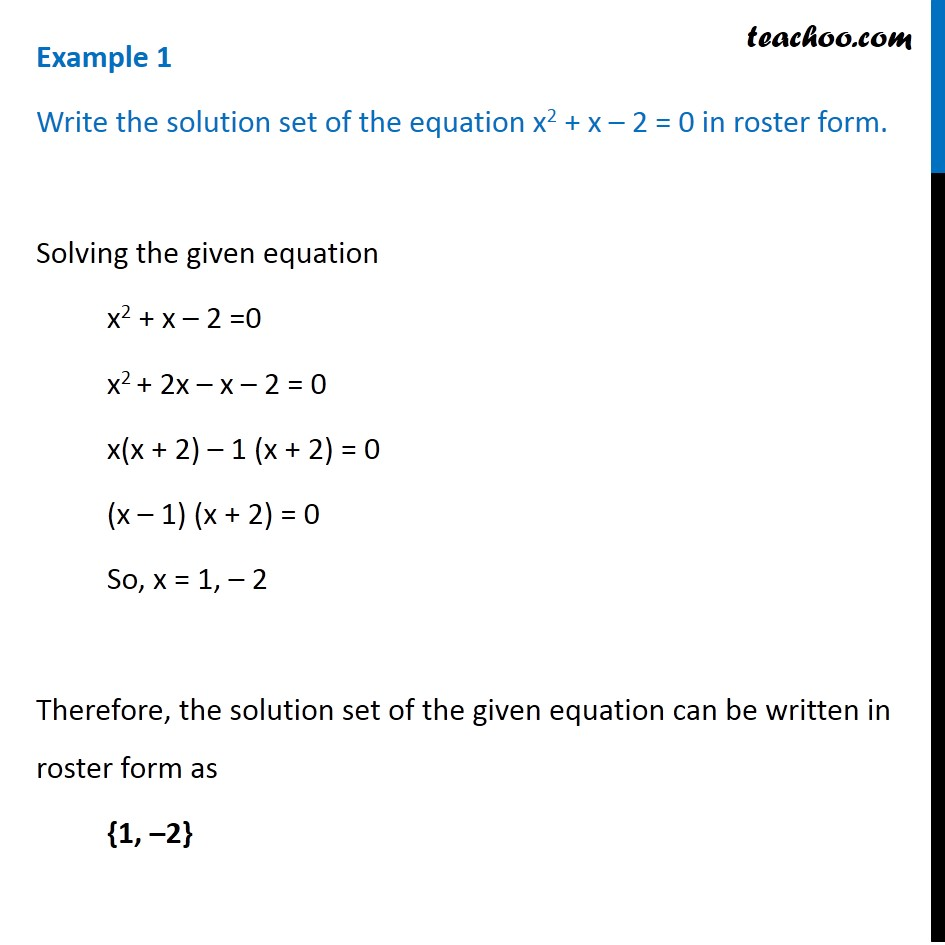1. Chapter 1 Class 11 Sets
2. Concept wise
3. Depicition of sets - Roster form

Transcript

Example 1 Write the solution set of the equation x2 + x – 2 = 0 in roster form. Solving the given equation x2 + x – 2 =0 x2 + 2x – x – 2 = 0 x(x + 2) – 1 (x + 2) = 0 (x – 1) (x + 2) = 0 So, x = 1, – 2 Therefore, the solution set of the given equation can be written in roster form as {1, –2}

Depicition of sets - Roster form